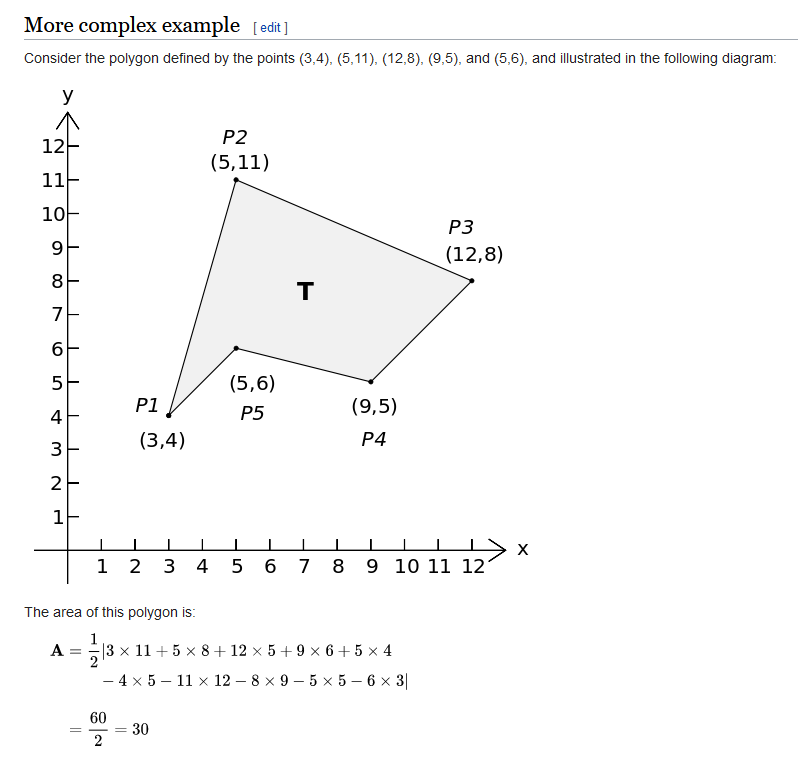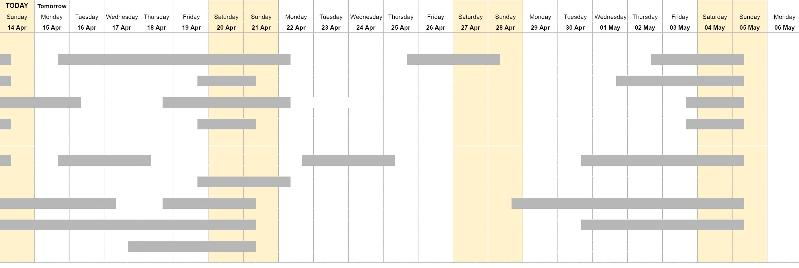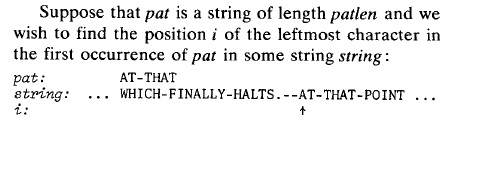Algorithms

4K

Solutions

4K

Contributors

An algorithm is a self-contained step-by-step set of operations to be performed. Algorithms exist that perform calculation, data processing, and automated reasoning. Starting from an initial state and initial input (perhaps empty), the instructions describe a computation that, when executed, proceeds through a finite number of well-defined successive states, eventually producing "output" and terminating at a final ending state. The transition from one state to the next is not necessarily deterministic; some algorithms, known as randomized algorithms, incorporate random input.

Share tech news, updates, or what's on your mind.
Hello,
First at all sorry for my bad English.
I would like to create a kind of lock with no internet connexion and a RTC inside.
The lock will open with a code 8 chars long. The chars can be from 0 to 9 and from A to D. (I use a 16 key keypad)
The main idea is to have a code generator that encrypt with this 14 chars (0-9 and A-D) a code that says to the lock : you will have to open from this date until this date. And because i want the code to work only with the serial of one lock the code have to be compatible only with the lock I create the code for.

So to resume :
I have a lock with a 16 keys that are 0-9 and A-D the last two are R for reset and V like run :)
The lock have a serial number could be 000 to 999
When I create the code the code have to contain on max 8 chars : the start date, end date and the serial of the lock.

I've tried some ideas like work with a base 14 or something like. Use a kind of offset from the a defined date that is the same on the lock and the generator and then use the difference from this date to create the start date. Use the serial of the lock to do a CRC so when the lock check the code if the CRC is not ok then it won't open.

Well as you see I'm getting lost so if you have a simple idea please help

Thanks a lot
0
In fact this are really three question:
1.) is grep -f memory friendly? (so could I avoid writing any python code)
2.) Does somebody have already a working solution for below problem?
3.) Could you make suggestions or review my code as soon as it starts working.

I'm trying to find a solution, that works for really huge files. (will not  use more RAM if files have more lines)
The problem: identify all lines, that exist in the first file, but don't exist
in the second one. In none of the files the same line can occur twice

Here a small example:

file1 (f1.txt)
aba
abb
abd
abf
one
two
three
four
five
six
seven

File2 (f2.txt)
abd
abe
eleven
four
eight
one
six

The expected result (order is not relevant)
aba
abb
abf
five
seven
three
two

I have already three approaches and an idea for the fourth one
1.) use grep -v -f f2.txt  f1.txt but I know nothing at all about memory consumption
2.) both files can be read into memory (so not suitable for huge files)
3.) the first file is huge the file fits into memory (so not suitable if the second file is huge)
4.) I'm working on it. In the moment the solution does not work as I didn't finish it. The idea es explained below

I have an idea of how to implement a solution, but wanted to know whether anybody has already written such code or knows of a standard solution.

My idea is to sort both files (the default linux sort is quite smart and ensures, that it works …
0
Suppose a heap file contains 100,000 records of 400 bytes each. Block size is 4096.  During processing, 2 records get added to the end of the file for every record deleted somewhere within the file.  This continues until the file has 200,000 active records.

a. How many disk blocks does the file currently occupy?
b. How many records have been deleted?
c. How percentage of the space within the file is not being used?
0
What can you tell me about Expert Systems?

I work for a technology company where there are hundreds of very smart engineers such as Electrical Engineers, Chemical Engineers and Manufacturing engineers.

They are all very smart and skilled. But the downside of every one is they are human.

I look at Supercomputers, like Summit, by IBM (200 Trillion computations per second), as well as Watson, which I know very little about, and I worry that Watson could wipe out small businesses like the one I work for.

How close are Expert Systems like Watson from performing completed designs, automatically? Let's say, for example, an integrated circuit.

I am curious to hear what a company that sees Watson as their true competition can do on a shoestring budget to harness their own engineers knowledge in a form that can be coalesced into a internal use only decision support system.

The sky's the limit on your theories...This is a wide open question, meant to tickle the mind.

Thanks
0
Try to get some smart loops in C/C++ below:
Assume that we have a series: 2, 3, 4, 8.  Want to get the different sums of all combinations, that is
s1=2+3, s2=2+4, s3=2+8, s4=3+4, s5=3+8, s6=4+8, s7=2+3+4, s8=3+4+8,s9=2+4+8, s10=2+3+4+8. How about number of elements are 50 instead 4?
Can any gurus shed some light to it?
0
How can I use LINQ to go through a list of Points using this formula?

I'm confident I know how to build a function for it but I really like LINQ and I believe it's a prime candidate for this calculation. I'm currently reading about it.

List<Point> PolyPoints = new List<Point>();0
Hi,

I looking for a measure of fragmentation, and occupancy of a calendar.

This is to assist in managing calendar events in order to reduce gaps between events and increase overall efficiency.

Attached is an example calendar, the grey bars are events. Each row is a resource. The events can sometimes be moved to different resources, and it can be helpful to quantify the benefit of a move.

What kinds of analysis techniques exist in this area?

Are there any analogies to other systems that could be helpful. eg if the calendar were a river, the bars islands, maybe efficiency might be the average flow rate of the river?

Thanks for your thoughts here.0
Hi,
I want to add the following algorithms to my ssh and sftp server.
aes128-ctr hmac-sha1
As per my knowledege aes128-ctr is something related to encryption and
hmac-sha1 is related to authentication.
I have a sftp server running on a machine.
I want it to support these two.
Does it need to be added to sftp server ?
or ssh ?
The machine is having openSSH 7.9

My aim is that ultimately anyone connecting to the sftp server these two algorithms should be available..

Thanks
0
Please explain Gamma Compression algorithms. How to write Gamma Compression algorithms for typical video and image processing system?  Please provide some detailed examples.  How to gain sufficient understanding to be able to write Gamma Compression in 'C' or MATLAB?
0
Hi friends,

I have some visualisation project where textual info is displayed on a colored background (that is not disputable). The background color is meant to give a mental shortcut to the value, and it could be basically any color in the 3 byte RGB space. Nevertheless the textual info needs to be easily readable against the background.

I've found some algorithms to generate contrasting colors from background color by inverting (XOR with #FF) the R, G and B bytes of the background color, some with improvements for R/G/B values near 128. Unfortunately these algorithms generate some color combinations which are (to be very, very euphemistic) more insulting to the eye than mere unergonomic .

What I need is some algorithm that produces contrasting, but eye-friendly companion foreground colors out of the values of the background color values. (I'd take ready-to-use code, too ;-)

Any idea ?
0
Is KPM the String-searching algorithm needed to give user a City Name search field?

I need to code a CityName input field which allows the user to type a few characters, then show the user the closest matches, in a list.

Can you break this out into what is generally needed for this algorithm to work? Is KPM a choice?

As you can see, I just need to get my arms around this, conceptually.

I would appreciate any guidance you can provide me.

Thanks
0
What are some common conversational terms used when discussing the BigO notation?

I think this wold be a good way to give me more exposure to learn the meaning of common terms used when discussing the BigO notation.

Please provide as many as come to mind...

Thanks
0
What performance consideration (for sorting) are there in .NET regarding various List Types? Arrays? Other?

What options are there when choosing a list type to use to hold large amounts of data? And what are the advantages of one of the other in a quick sort-time is required?

And, besides sorting, where would performance be a consideration?

Thanks
0
The big terms of BigO Notation

I want to drill down into BigO, but hope to do so from a usefulness perspective. So, regarding making low-level algorithms more performant, for example. Or, from a memory management view...

Suggested topics to read up on? And a site where I can read this?

Thanks
0
Hi all,

I do want to store  "directory path" as a  key and corresponding value as a value. The key would be 1024.

If I take std::map, I guess it would be performance hit with huge number of entries.

It has to maintain this ds inside kernel module. What is the best ds to implement this in C++ 11?

Thanks,
Bvm.
0
What does       O(n2) algorithm mean? and How to test it to show running time?
0
Algorithm question
Suppose an O(n3) function takes 10 seconds to work on a dataset of size n=400.  How long would we expect this function to execute on a dataset of size n=800?
0
I need some help coming up with a present value formula to calculate what we call in our industry the true interest cost of debt or TIC.   In the attached file is the calculation produced by software that we use however it does not provide formulas.   I would like to come up with a spreadsheet that I can use to calculate TIC on my own.   About the spreadsheet that I have provided.   At the top is the solution to the cash flows below.   The dated date is the date that interest begins on the loan the TIC is the effective borrowing rate or the rate necessary to discount the amounts payable on the respective principal and interest payment dates to the purchase price (loan amount) received assuming compounded semi-annually.   The first column is the dates payments are made, the second column is the amount of debt paid each semi period and the third column is the discount column calculated by the software to come up with the TIC (discount rate) that equals the target amount above of \$10,513,798.96.  I would like to setup a spreadsheet that will allow me to calculate on my on the TIC.  Meaning if I know the target amount, Dated Date, and semi-annual cash flows the spreadsheet will calculate the TIC that discounts those cash flows so that they equal the target amount.  I have tried using the IRR function but it did not work for me.  Hoping to get other ideas how to set this up using this example to check that it works by trying to recreate the answer.  I hope this make sense but …
0
Hi,

I am practicing some algorithm to merge 2 existing arrays.
Here are the requirements I created:
1. no Linq allowed.
2. no concat, copyTo. Array.Sort() method allowed.
3. result should be sorted by ascending order.

I actually completed the coding, but there must be much better solution.
I also had to create Sort method to sort the result array.
Is there anyway I can sort while merging?

Currently I am Merging and then Sorting.

static void Main(string[] args)
{

int[] a = { 1, 2, 3 };
int[] b = { 1, 2, 3 };

int[] result = Sort(MergeTwoArrays(a, b));
foreach (var item in result)
{
Console.Write(item+ " ");
}

}

public static int[] MergeTwoArrays(int[] a, int[]b)
{
int aLength = a.Length;
int bLength = b.Length;
int totalLength = aLength + bLength;
int[] c = new int[totalLength];

for (int i = 0; i < a.Length; i++)
{
c[i] = a[i];
}
int k = a.Length;
for (int i = 0; i < b.Length; i++)
{
c[k] = b[i];
k++;
}
return c;
}

public static int[] Sort(int[] a)
{
int i, j, temp, minIndex;
for (i = 0; i < a.Length; i++)
{
minIndex = i;

0
I've been using the Azure ML Studio and it is great.

I get the basics of what ML is and what it does. But now I'd like to learn it in more detail - understand the deeper issues, the math behind it, what the different algorithms do and so on.

But I don't know where to go for this. Most of the introductions to ML use Python and TensorFlow. Ideally I'd like to stick to Azure ML Studio but I just can't find anything that tells me about the theory of ML rather than how to use Azure ML Studio.

I'm talking about books or courses - I think ideally a book but either would be fine.

This book might be promising:

Predictive Analytics with Microsoft Azure Machine Learning 2nd Edition Paperback – 19 Aug 2015
by Valentine Fontama (Author), Roger Barga (Contributor), Wee Hyong Tok (Contributor)

But I'm concerned it doesn't give the background - the reviews seem to indicate this.

Let me know if you have read a good book or completed a good course that explains the math of ML and would be something I could use with the Azure ML Studio.

Thanks!
0
Need to search find closest match in array of strings

I have a static list of about 500 strings containing things like:

VS Credit Voucher Proc-CR Trans 2
VS Credit Voucher Proc-OB Prepaid Trans 2

but am reading from OCR and get the strings from the faxed reports looking like:

VS Credit Voucher Proc-CR Trans 2
VS Crect Voucher Proc-OBPrepaid Trar 2

I need to do a lookup for the best match for each as it appears in the in the static list.

And of course, there needs to be a threshold where NO MATCH is a possibility.

How shall I store the static list? How can I do a search in the list that is resource efficient?

I would sort that list of 500, clearly. But what are the mechanics of the lookup?

I am writing a C# Win Forms (64 bit) application and could include a database, if I could include that into my EXE, to avoid a distinct installation step.

What search algorithm?

Thanks.
0
I am considering whether the starting position for the pattern vis-a-vis the searched text,  (i.e. the LAST CHARACTER of the pattern be aligned) ought to initially coincide with the first appearance of that LAST CHARACTER in the searched Text, *provided that appearance is equal to or more than the length of the Pattern*, since otherwise the index would have occurred too early in the sequence to be valid.

Consider this illustration from the B-M paper :The B-M algo would 'mismatch' the final T from the 'pat' with the F from the 'string', and move the pattern past the F, to align the A with the I. Whereas the first contending T in the pattern and the string is at index 17 in the string, rather than the standing comparison being done at index 7.
0
Are there any comments anyone would like to make that would flatter or support any particular approach to String search methodology and algorithms ? Are there any algorithms which can be applied to several search conditions and requirements ? All comments welcomed. Thanks, k.
0
Implement an algorithm as method to take a BST and convert it to a mirror tree where left and right subtrees are interchanged.
and print BST each level in separate line
All coding should be in Python
0
Dear experts,

I have learnt about GeoGebra app while learning about One note from one of the Youtube videos.

I have tried it and i see that produces good charts. But it does not the values used in the x variable to calculate the Y value.

In the attached screen dump I have shown the table calculated by me. the function graph has been copy[-pasted from Geogebra.

CAn you someone please guide me to a app/url which plot the function and shows the table.

Thank you
WhatsApp-Image-2018-04-13-at-07.07..jpeg
0

Algorithms

4K

Solutions

4K

Contributors

An algorithm is a self-contained step-by-step set of operations to be performed. Algorithms exist that perform calculation, data processing, and automated reasoning. Starting from an initial state and initial input (perhaps empty), the instructions describe a computation that, when executed, proceeds through a finite number of well-defined successive states, eventually producing "output" and terminating at a final ending state. The transition from one state to the next is not necessarily deterministic; some algorithms, known as randomized algorithms, incorporate random input.

Top Experts In
Algorithms
Monthly